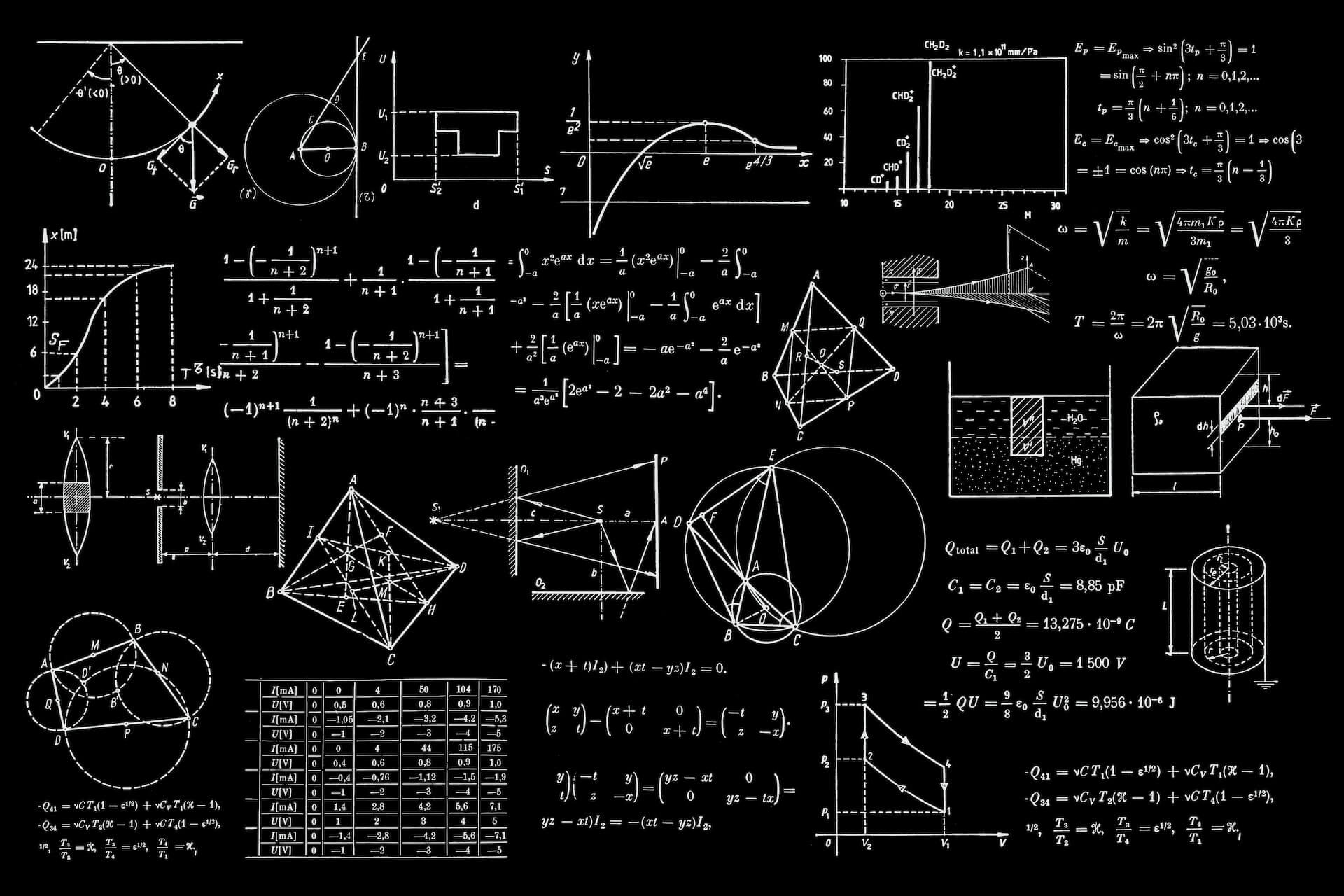# Unit Circle and Trigonometric Values | Example | FAQs

December 22, 2022Calculus, pre-calculus, and trigonometry frequently refer to and involve the unit circle in their applications.

A unit circle is a mathematical tool to simplify the application of trigonometric functions and angles. By understanding the unit circle, we can quickly and efficiently solve problems that would typically require much calculation.

## What is a unit circle?

A circle with a radius of one unit and a center at the origin is referred to as a unit circle. A unit circle is the location of a point one unit away from a fixed point. When working with trigonometric functions and angle measurements, the unit circle is a valuable tool that makes reference much simpler.

We can use the unit circle to apply the Pythagorean Theorem. Remember that the Pythagorean Theorem is used to locate a right triangle’s missing side. Check Pythagorean Theorem Worksheet.

The triangle formed in the unit circle image to the right is a right triangle. Where the radius (r) is the hypotenuse, the side lengths of x and y are the legs.

## Unit Circle and Trigonometric Values

A unit circle can compute trigonometric identities and their principal angle values. Cosine is the x-coordinate, and sine is the y-coordinate in the unit circle. Let’s now calculate the values for θ = 0° and θ = 90º.

### Sin, Cos, Tan at 0°

The x-coordinate and y-coordinate are 1 and 0, respectively, for θ = 0°. As a result, cos0º = 1, sin0º = 0, and tan0º = 0

### Sin, Cos, Tan at 90°

Let’s take a look at another 90º angle. Here, cos90º = 0, sin90º = 1, and tan 90° are undefined.

### Special Angles: 30°, 45°, and 60°

Additionally, let’s use this unit circle to calculate the values of crucial trigonometric functions, values of θ such as 30º, 45º, and 60º.

 $$sin$$ $$cos$$ $$tan$$ or $$\dfrac{sin}{cos}$$ $$0º$$ $$30º$$ $$45º$$ $$60º$$ $$90º$$ $$0$$ $$\dfrac{1}{2}$$ $$\dfrac{\sqrt{2}}{2}$$ $$\dfrac{\sqrt{3}}{2}$$ $$1$$ $$1$$ $$\dfrac{\sqrt{3}}{2}$$ $$\dfrac{\sqrt{2}}{2}$$ $$\dfrac{1}{2}$$ $$0$$ $$0$$ $$\dfrac{\sqrt{3}}{3}$$ $$0$$ $$1$$ undefined

## How to Use the Unit Circle:

The best way to get comfortable using the unit circle is by solving some practice questions.

### Example:

To find sin 4π/3 first, Identify The Quadrant:

We only need to determine which quadrant we are in to determine if our answer will be positive or negative because we are working with sine. Since:

3π/2 > 4π/3 > π

This places us in the third quadrant. We will therefore get a negative response because sine provides us with the y coordinate, and we are in the third quadrant.

Now solve:

The following stage is easy since we can solve this problem quickly utilizing the information we have memorized.

Answer: sin 4π/3 = – $$\dfrac{\sqrt{3}}{2}$$

## How to Compute Unit Circle With Tangent Values?

The trigonometric circle of the tangent function is another name for the unit circle with a tangent. It provides the trigonometric function “tan” values for several standard angles that range from 0° to 360°.

## How to memorize the unit circle?

So all silver tea cups are in the first through fourth quadrants. All of the functions in the first quadrant are positive.

• Silver-Sine in the second quadrant and its inverse positive
• Tea-Tan in the third quadrant, with exclusively positive reversals.
• Cups in the fourth quadrant, Cos, and only the positive inverse

This is handy when you try to add an angle to another within a trigonometric function.

For instance, sine and its inverse provide a positive result, as in sin(90+X) = Cos (X). Any other function will result in a negative value. Therefore Cos(90+X) = – sin(X).

Following the property, any angle in these quadrants will have either positive or negative values.)

Now fully get that the sine axis is the Y axis and the cosine axis is the X axis. Any angle X will therefore have a coordinate of the form (cosX, sinX), and when plotting, remember that you can adhere to the standard guidelines (for instance, the point in the second quadrant will be of the type (- cosX, sinX). Therefore, the coordinate reasoning is the same.

Now all you need to do is plot the points on the graph and understand that they will eventually converge to form a circle. This is the easiest way to memorize a unit circle.

## What are the unit circle radians?

When the arc it makes on the circle’s circumference is equal to the radius of the circle, the central angle of the circle is measured in radians. The radius on the unit circle is 1. So the angle with an arc length of one radian intersects the circle.